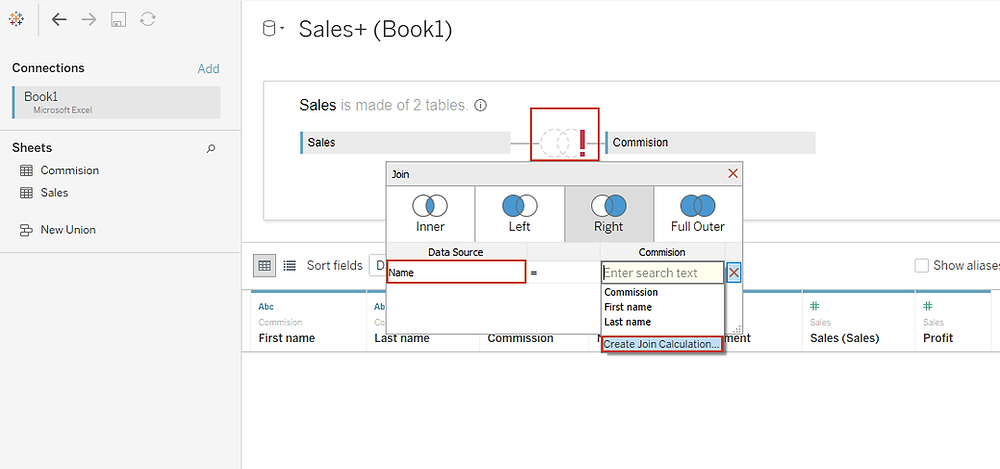Search
• Bernard

# Tableau Join CalculationData joining is the technique of combining two or more tables together using a common field. The results of joining tables is a virtual table with additional columns added to the primary data source. The fields used to create the data join MUST be of the same data type.

Sometimes, the fields required to join tables may be different (mismatched), requiring you to create a Tableau join calculation. A good example of mismatched fields is in the example below where the fields required to create the join occur as a single field in one table while on the other table exist as two fields.(To join these two tables, I will need to create a common field between the two tables by simply combining the ‘Firsts name’ and ‘Last name’ in the second table to match the first table. This can be done using a join calculation)

Once connected to the data source, on the physical layer of the new Tableau data source (launched in Tableau 2020.2) you can join the tables using Tableau join calculation as shown.Executing this we have.(This table is a proof that our Tableau join calculation was successful)

## Spatial Join Calculations

When doing geographical analysis, you can use Tableau join calculations to join spatial data with non-spatial data that contains latitude and longitude fields.

To join Spatial data with non spatial data, you use the function MakePoint(Latitude, Longitude) - which converts data from latitude and longitude fields into spatial objects.

Note: The non-spatial data to be used in the join MUST have latitude and longitude fields.

To perform spatial join calculation, connect the spatial file first, add the non-spatial file and drag the table to the canvas on the physical layer of the Tableau data source.

On the spatial file choose 'Geometry' while on the non-spatial data choose 'Create Join Calculation...'